A Source Book in Mathematics, 1200-1800

Contents:
Author: René Descartes  | Date: 1637

Show Summary
Mathematics

4 DESCARTES.

# The Principle of Nonhomogeneity

All problems in geometry can easily be reduced to such terms that a knowledge of the lengths of certain straight lines is sufficient for their construction.

Just as arithmetic consists of only four or five operations, namely, addition, subtraction, multiplication, division, and the extraction of roots, which may be considered a kind of division, so in geometry, to find required lines it is merely necessary to add or subtract other lines; or else, taking one line which I shall call the unit in order to relate it as closely as possible to numbers, and which can in general be chosen arbitrarily, and having given two other lines, to find a fourth line which shall be to one of the given lines as the other is to the unit (which is the same as multiplication); or, again, to find a fourth line which is to one of the given lines as the unit is to the other (which is equivalent to division); or, finally, to find one, two, or several mean proportionals between the unit and some other line (which is the same as extracting the square root, cube root, etc., of the given line). And I shall not fear to introduce these arithmetical terms into geometry, for the sake of greater clarity.

For example, let AB [Fig. 1] be taken as the unit, and let it be required to multiply BD by BC. I have only to join the points A and C, and draw DE parallel to CA; then BE is the product of BD and BC.

[figure table]

Fig. 1

If it be required to divide BE by BD, I join E and D, and draw AC parallel to DE; then BC is the result of the division.

Or, if the square root of GH [Fig. 2] is desired, I add, along the same straight line, FG equal to the unit; then, bisecting FH at K, I describe the circle FIH about K as a center and draw from G a perpendicular and extend it to I, and GI is the required root. I do not speak here of cube root, or other roots, since I shall speak more conveniently of them later.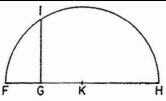Fig. 2

Often it is not necessary thus to draw the lines on paper, but it is sufficient to designate each by a single letter. Thus, to add the lines BD and GH, I call one a and the other b, and write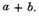Then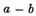will indicate that b is subtracted from a; ab that a is multiplied by b; a/b1 that a is divided by b; aa or a2 that a is multiplied by itself; a3 that this result is multiplied by a, and so on, indefinitely. Again, if I wish to extract the square root of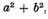I write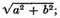if I wish to extract the cube root of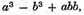I write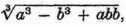2 and similarly for other roots. Here it must be observed that by a2, b3, and similar expressions, I ordinarily mean only simple lines, which, however, I name squares, cubes, etc., so that I make use of the terms employed in algebra.

It should also be noted that all parts of a single line should as a rule be expressed by the same number of dimensions, when the unit is not determined in the problem. Thus, a3 contains as many dimensions as abb or b3, these being the component parts of the line which I have called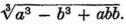It is not, however, the same thing when the unit is determined, because it can always be understood, even where there are too many or too few dimensions; thus, if it be required to extract the cube root of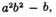we must consider the quantity a2b2 divided once by the unit, and the quantity b multiplied twice by the unit.

Finally, so that we may be sure to remember the names of these lines, a separate list should always be made as often as names are assigned or changed. For example, we may write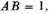that is AB is equal to 1;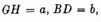and so on.3

If, then, we wish to solve any problem, we first suppose the solution already effected,4 and give names to all the lines that seem needful for its construction—to those that are unknown as well as to those that are known. Then, making no distinction between known and unknown lines, we must unravel the difficulty in any way that shows most naturally the relations between these lines, until we find it possible to express a single quantity in two ways. This will constitute what we call an equation, since the terms of one of these two expressions are equal to those of the other. And we find as many such equations as there are supposed to be unknown lines; but if, after considering everything involved, so many cannot be found, it is evident that the question is not entirely determined. In such a case we may choose arbitrarily lines of known length for each unknown line to which there corresponds no equation.

If there are several equations, we must use each in order, either considering it alone or comparing it with the others, so as to obtain a value for each of the unknown lines; and so we must combine them until there remains a single unknown line which is equal to some known line, or whose square, cube, fourth power, fifth power, sixth power, etc., is equal to the sum or difference of two or more quantities, one of which is known, while the others consist of mean proportionals between unity and this square, or cube, or fourth power, etc., multiplied by other known lines. I express this as follows: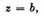or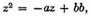or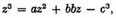or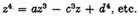That is, z, which I take for the unknown quantity, is equal to b; or, the square of z is equal to the square of b diminished by the square of z, plus the square of b multiplied by z, diminished by the cube of c; and similarly for the others.

Thus, all the unknown quantities can be expressed in terms of a single quantity, whenever the problem can be constructed by means of circles and straight lines, or also by conic sections, or even by some other curve only one or two degrees more composed.

But I shall not stop to explain this in more detail, because I should deprive you of the pleasure of mastering it yourself, as well as of the advantage of training your mind by working over it, which is in my opinion the principal benefit to be derived from this science. Because I find nothing here so difficult that it cannot be worked out by any one at all familiar with ordinary geometry and with algebra, who will consider carefully all that is set forth in this treatise.

That is why I shall content myself with the statement that if the student, in solving these equations, does not fail to make use of division wherever possible, he will surely reach the simplest terms to which the problem can be reduced.

And if it can be solved by ordinary geometry, that is, by the use of straight lines and circles traced on a plane surface, when the last equation shall have been entirely solved there will remain at most only the square of an unknown quantity, equal to the product of its root by some known quantity, increased or diminished by some other quantity also known.5 Then this root or unknown line can easily be found. For example, if I have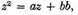I construct [Fig. 3] a right triangle NLM with one side LM, equal to b, the square root of the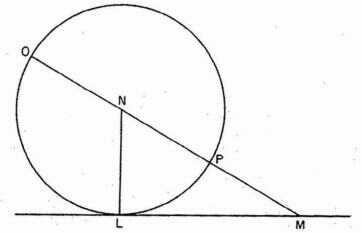Fig. 3

known quantity bb, and the other side, LN, equal to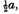that is to half the other known quantity which was multiplied by z, which I suppose to be the unknown line. Then prolonging MN, the hypotenuse [la baze] of this triangle, to O, so that NO is equal to NL, the whole line OM is the required line z. It is expressed in the following way: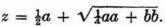But if I have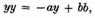where y is the quantity whose value is desired, I construct the same right triangle NLM, and on the hypotenuse MN lay off NP equal to NL, and the remainder PM is y, the desired root. Thus I have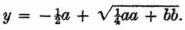In the same way if I had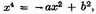PM would be x2 and I should have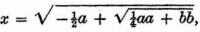and so for other cases.

Finally, if I have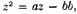I make NL equal to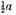and LM equal to b as before; then [Fig. 4], instead of joining the points M and N, I draw MGR parallel to LN, and with N as a center describe a circle through L cutting MGR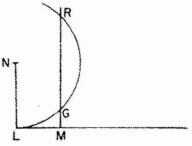Fig. 4

in the points G and R; then z, the line sought, is either MG or MR, for in this case it can be expressed in two ways, namely,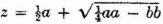and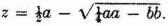And if the circle described about N and passing through L neither cuts nor touches the line MGR, the equation has no root, so that we may say that the construction of the problem is impossible.6

These same roots can be found by many other methods. I have given these very simple ones to show that it is possible to construct all the problems of ordinary geometry by doing no more than the little covered in the four figures that I have explained. This is one thing which I believe the ancient mathematicians did not observe, for otherwise they would not have put so much labor into writing so many books in which the very sequence of the propositions shows that they did not have a sure method of finding them all, but rather gathered those propositions on which they had happened by accident.

1 Descartes writes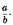2 Descartes writes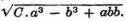3 Descartes writes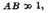etc.; see Selection II.8, note 3.

4 This is the "analysis" of Pappus, see Selection II.5.

5 Hence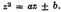6 Descartes here follows the common practice of his day, which considered only the types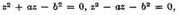and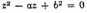of quadratic equations, ignoring the type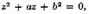since it has no positive roots (a is a segment, hence positive). Only much later (Newton) did mathematicians begin to associate coordinates with negative numbers. All coordinates in Descartes are positive. The name "coordinate" does not appear in Descartes; this term is due to Leibniz.

Contents:

### Related Resources

René Descartes

Title: A Source Book in Mathematics, 1200-1800

Select an option:

## Email Options

Title: A Source Book in Mathematics, 1200-1800

Select an option: